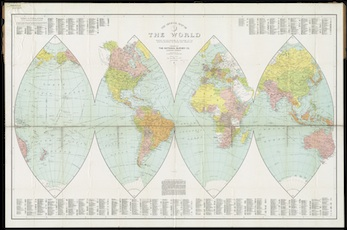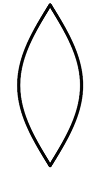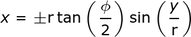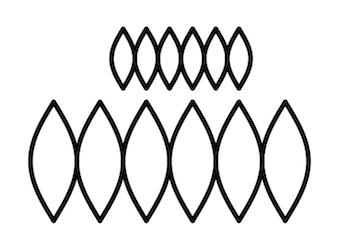Math Courses / Course / Chapter

# Similar Solids: Definition, Properties, Area & Volume

Instructor: Gerald Lemay

Gerald has taught engineering, math and science and has a doctorate in electrical engineering.

The concept of similarity goes beyond similar triangles and includes similar solids. In this lesson the properties of similar solids is examined for the sphere and then extended for all other solids.

## Similar Solids

Resembling a collage of sliced orange peels, maps have advantages over globes. For example, a map is foldable, making it easy to carry. A globe, well, is more difficult to hike with; just ask the fictional explorer, Henri du Math.The equation for the surface area of a sphere can be derived by analyzing slices. In this lesson, these results help explain what happens to the properties of any solid when it is transformed into a similar solid. Henri would transform an orange slice into a snack.

## Definitions

Making a scaled version of a solid, creates a similar solid. The scaling happens by multiplying each defining dimension by the same number. This number is the scale factor α and for the sphere, the radius r is the defining dimension. For other solids, the defining dimension(s) are lengths of sides, radii and heights. But it is still the same procedure:

• determine the defining dimension(s) for the solid
• multiply each of those dimensions by a scale factor α

Creating a similar solid has predictable effects on the properties of surface area and volume.

## Surface Area of a Sphere

Let's continue with the global map. The ''orange slice'' has a technical name. It's called a gore and resembles a pointed ellipse. The shape reminds Henri of his trusty canoe.Imagine the bottom tip at the origin where both x and y are zero. We are at the south pole of the globe. Traveling to the north pole is half the circumference of the globe. Half the circumference is half of 2π r or just π r. Thus, the values for y are from 0 to π r.

The sine of 0 is zero and the sine of π is zero. The gore's x value is zero at two places: y = 0 and y = π r. Consider sin(y/r). When y is zero, sin(0/r) is zero. When y is π r, sin(π r/r) = sin(π) = 0. The maximum width of the gore is at the ''equator'' where the y-axis midpoint is π r/2. Meaning sin(y/r) is sin(π r/(2r)) = sin(π/2) = 1. Great! sin(y/r) works well in the equation for x.

The larger the r, the larger the sphere and the larger the gore's width. The gore subtends an angle φ that relates the width of the gore to the radius of the circle through the tangent. The tangent of φ/2 is the half the width of the gore divided by r. Thus, at the midpoint of y, the half-width is r tan(φ/2). Combining this with sin(y/r):Why the plus/minus? To get the full width, this equation is evaluated twice; once for the plus and once for the minus.

To find the area of one gore, integrate this function over y. This gives 4 r2 tan(φ/2). In terms of n gores, φ is 2π/n meaning φ/2 is π/n. Thus, the total surface area of the sphere with n gores is A = 4 nr2 tan(π/n).

As n gets larger, π/n gets smaller, and for these small values, π/n approximates tan(π/n). Thus, as n get larger, A = 4 nr2 tan(π/n) becomes A = 4 nr2 (π/n). The n's cancel leaving A = 4π r2 (the equation for the surface area of a sphere).

Henri enjoyed the journey from the south pole to the north pole but is glad to have finally arrived at the sphere surface area equation, A = 4π r2.

### Scaling

A sphere with radius 2 has been mapped with six gores. Below it is a similar sphere with radius 4:A rectangle with length 2π r and width π r would fit over a gore map. The smaller sphere with r = 2 has length 2π r = 2π(2) = 4π and width π r = π2 = 2π. The area of the rectangle is length times width = (4π)(2π) = 8 π^2. For the larger sphere of radius r = 4, the area is (8π)(4π) = 32π; four times greater surface area.

Thus, scaling by α increases the surface area by a factor of α^2.

This is important to Henri because scaling his canoe by α means α^2 more surface area and α^2 more paint to cover his canoe.

To unlock this lesson you must be a Study.com Member.

### Register to view this lesson

Are you a student or a teacher?

Back

### Resources created by teachers for teachers

Over 30,000 video lessons & teaching resources‐all in one place.Video lessonsQuizzes & WorksheetsClassroom IntegrationLesson Plans

I would definitely recommend Study.com to my colleagues. It’s like a teacher waved a magic wand and did the work for me. I feel like it’s a lifeline.

Jennifer B.
TeacherCreate an account to start this course today
Used by over 30 million students worldwide